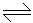# Chemical Engineering - Chemical Reaction Engineering - Discussion

5.

For the reversible reaction A2B, if the equilibrium constant K is 0.05 mole/litre; starting from initially 2 moles of A and zero moles of B, how many moles will be formed at equilibrium ?

 [A]. 0.253 [B]. 0.338 [C]. 0.152 [D]. 0.637

Explanation:

No answer description available for this question.

 Balemual Kumelachew said: (Oct 13, 2017) Thank you.

 Sachin said: (Aug 3, 2018) Explain the Solution please.

 Anju Singh said: (Feb 28, 2019) k = [product]^n/[reactant]. A = 2B 2 ----------- 0 2-x ----------- 2x And total no of moles = 2x+2-x = 2+x. K=(2x/2+x)^2/[(2-x)/(2+x)] put the value of k solved the equation. x=.2238. No of moles formed = 2* x = .4476. So, option (B) is correct.